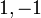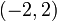# Difference between revisions of "Element structure of special linear group:SL(2,R)"

## Contents

View element structure of particular groups | View other specific information about special linear group:SL(2,R)

This article aims to discuss the element structure of special linear group:SL(2,R).

## Conjugacy class structure

To deduce this from element structure of special linear group of degree two over a field, we need to use the following facts, about$\R$, the field of real numbers:

• The group$\R^*/(\R^*)^2$ is cyclic of order two, with representatives$1, -1$.
• The only separable quadratic extension of$\R$ is the field of complex numbers, obtained by adjoining a square root of -1.
• Further, the algebraic norm of any nonzero complex number is a positive real number, and in particular, it is a square. Thus,$\R^*/N(\mathbb{C}^*)$ has size two.
Nature of conjugacy class Eigenvalues Characteristic polynomial Minimal polynomial What set can each conjugacy class be identified with? (rough measure of size of conjugacy class) What can the set of conjuacy classes be identified with (rough measure of number of conjugacy classes) What can the union of conjugacy classes be identified with? Semisimple? Diagonalizable over$\R$? Splits in$SL_2$ relative to$GL_2$?
Diagonalizable over$\R$ with equal diagonal entries, hence a scalar$\{ 1,1 \}$ or$\{ -1,-1\}$$(x - a)^2$ where$a \in \{ -1,1 \}$$x - a$ where$a \in \{ -1,1\}$ one-point set two-point set two-point set Yes Yes No
Not diagonal, has Jordan block of size two$1$ (multiplicity 2) or$-1$ (multiplicity 2)$(x - a)^2$ where$a \in \{ -1,1 \}$ Same as characteristic polynomial  ? four-point set, two for eigenvalue 1, two for eigenvalue -1  ? No No Both the$GL_2$-conjugacy classes split into two pieces.
Diagonalizable over$\mathbb{C}$ but not over$\R$. Must necessarily have no repeated eigenvalues. Pair of conjugate elements in$\mathbb{C}$ of modulus 1$x^2 - ax + 1$,$-2 \le a \le 2$ Same as characteristic polynomial  ? direct product of the open interval$(-2,2)$ with a two-point set  ? Yes No each$GL_2$-conjugacy class splits into two$SL_2$-conjugacy classes.
Diagonalizable over$K$ with distinct (and hence mutually inverse) diagonal entries$\lambda, 1/\lambda$ where$\lambda \in \R \setminus \{ 0,1,-1 \}$$x^2 - (\lambda + 1/\lambda)x + 1$ Same as characteristic polynomial  ?$\R \setminus [-2,2]$  ? Yes Yes No
Total NA NA NA NA  ?  ?  ?  ?  ?